## Thursday, December 23, 2010

### 2011

2011 is a prime number.

2011 has the representation 211 - 37.

2011 is the sum of 11 consecutive primes: 2011 = 157 + 163 + 167 + 173 + 179 + 181 + 191 + 193 + 197 + 199 + 211.

2011 is a number such that first reversing the digits and then squaring equals the result of first squaring and then reversing the digits: 11022 =1214404 and 20112 = 4044121.

28 is 2011 in base 5.

The sum of the digits of 2011 is equal to the number of digits: 2 + 0 + 1 + 1 = 4. The digit sum is a square and a power of 2.The next prime date from now (month, day, and year are primes) is 2/2/2011. Note that 222011 is a prime.

In the Aeon Flux television series and film (2005), 99 percent of the world's population is wiped out by a mysterious virus in the year 2011.

The Peace Corps marks its 50th anniversary in the year 2011. It is also the 5oth anniversary of the first manned mission to space.

2011 is the 100th anniversary of the launch of the Titanic on May 31, 1911.

2011 is designated the International Year of Chemistry.## Wednesday, December 22, 2010

### 982

982 = 2 x 491.

982 is the number of integer partitions of 39 into distinct parts.

982 is the number of ways to place four non-attacking queens on a 7 x 7 board.

Written backwards, 982 is the square 289.NGC 982 is a spiral galaxy in the constellation Andromeda.

## Tuesday, December 21, 2010

### 165

165 = 3 x 5 x 11.

165 is 10100101 in base 2 (binary).

165 is the number of integer partitions of 26 into distinct parts.

165 is the ninth tetrahedral number.

165 is the sum of the divisors of the first 14 integers.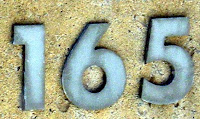The Tahoe Rim Trail is a 165-mile hiking trail around Lake Tahoe.

Source: WolframAlpha

## Monday, December 20, 2010

### 899

899 = 29 x 31. It is the product of two successive primes and the product of twin primes.

Any number consisting of an 8 followed by an even number of nines has prime factors 3 x (10n/2) + 1 and 3 x (10n/2) - 1, where n is the number of nines: 899 = 3(101) + 1) x 3(101) - 1 = 31 x 29. Similarly, 8999999 = 2999 x 3001.

899 is the smallest number whose sum of digits is 26.

899 is a number that can't be expressed as the sum of a prime number and a Fibonacci number.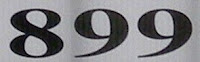The Benneli TNT 899 Sport is an Italian motorcycle.

## Friday, December 17, 2010

### 377

377 = 13 x 29.

377 has two representations as a sum of two squares: 377 = 42 + 192 = 112 + 162.

377 is the fourteenth Fibonacci number. It is the largest known Fibonacci number whose digits are all prime.

377 is the hypotenuse of two primitive Pythagorean triples: 3772 = 1352 + 3522 = 1522 + 3452.

377 is the sum of the squares of the first six primes: 377 = 22 + 32 + 52 + 72 + 112 + 132.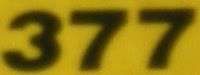The Aero Spacelines B-377 SG Super Guppy is a four-engine oversize-cargo freighter aircraft.

## Thursday, December 16, 2010

### 1196

1196 = 22 x 13 x 23.

1196 is the one thousandth non-prime number.

1196 is the number of lines through exactly two points of a 9 x 9 grid of points.

1196 is 14241 in base 5 and 616 in base 14.

1196 is the number of assymetric polyominoes made up of 9 cells.NGC 1196 is a galaxy in the constellation Eridanus, discovered by John Herschel in 1835.

## Wednesday, December 15, 2010

### 359

359 is a prime number. It is a Sophie Germain prime because 2 x 359 + 1 = 719, a prime.

359 is a number that cannot be written as a sum of three squares.

359 = -1 + 23 x 45.

The 359th, 360th, and 361st decimal digits of pi are 360.

359 has a base 3 representation (111022) that ends with its base 7 representation (1022), which is also true for 357 and 358.In the Star Trek imaginary universe, the Battle of Wolf 359 was the Federation's first major battle against a group of cyborgs.

## Tuesday, December 14, 2010

### 173

173 is a prime number. It is a Sophie Germain prime because 2 x 173 + 1 = 347, which is also prime.

173 has a representation as a sum of two squares: 173 = 22 + 132. It is the sum of the squares of two Fibonacci numbers, 2 and 13.

173 is the hypotenuse of a primitive Pythagorean triple: 1732 = 522 + 1652.

173 is the only prime whose sum of cubed digits equals its reversal: 13 + 73 + 33 = 371.

173 is the largest known prime whose square (29929) and cube (5177717) have no digits in common.

173 = 1 + 22 + 23 + 25 + 27, where the exponents are consecutive primes.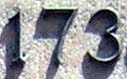In the 1993 movie The Sandlot, The Beast (a ferocious dog) is said to have eaten 173 guys.

## Monday, December 13, 2010

### 21

21 = 3 x 7.

21 is 10101 in base 2 (binary) and 111 in base 4.

21 has a unique representation as a sum of three squares: 21 = 12 + 22 + 42.

21 is the smallest number with five representations as a sum of three primes: 21 = 2 + 2 + 17 = 3 + 5 + 13 = 3 + 7 + 11 = 5 + 5 + 11 = 7 + 7 + 7.

21 is the eighth Fibonacci number.

21 is the fifth Motzkin number.

21 is the sixth triangular number.21 is the total number of spots on a normal die.

Source: Phillips, R. 2004. Numbers: Facts, Figures and Fiction. Badsey Publications.

## Friday, December 10, 2010

### 364

364 = 22 x 7 x 13.

364 has a unique representation as a sum of three squares: 364 = 22 + 62 + 182.

364 is the twelfth tetrahedral number.

364 is 111111 in base 3.

The first two digits of 364 are the product of the first two primes to the power of the first prime and the last two digits are the first prime to the product of the first two primes: 36 = (2 x 3)2 and 64 = 2(2 x 3).Asteroid 364 Isara was discovered in 1893.

## Thursday, December 9, 2010

### 954

954 = 2 x 32 x 53.

954 has a representation as a sum of two squares: 954 = 152 + 272.

954 is the smallest number n such that if you subtract its reverse from it, you get a number with the same digits as n: 954 - 459 = 495.

954 is 676 in base 12 and 585 in base 13.Steak 954 is a steakhouse in Fort Lauderdale, Florida.

## Wednesday, December 8, 2010

### 461

461 is a prime number.

461 has a representation as a sum of two squares: 461 = 102 + 192.

461 and 463 form a twin prime pair.

461 is the hypotenuse of a primitive Pythagorean triple: 4612 = 2612 + 3802.

461 is the largest number for which ((102n) + 1)/101 is known to be a prime number.Eric Clapton recorded a solo album titled 461 Ocean Boulevard.

## Tuesday, December 7, 2010

### 565

565 = 5 x 113.

565 has two representations as a sum of two squares: 565 = 62 + 232 = 92 + 222.

565 is the hypotenuse of two primitive Pythagorean triples: 5652 = 2762 + 4932 = 3962 + 4032.

565 is 474 in base 11.

565 is the sum of three consecutive primes: 565 = 181 + 191 + 193.in the year 565, St. Columba reported seeing a monster in Loch Ness, Scotland.

Source: WolframAlpha

## Monday, December 6, 2010

### 942

942 = 2 x 3 x 157.

942 is 666 in base 12.

942 is the smallest number whose cube contains five 8s: 9423 = 835,896,888.

942 is the sum of four consecutive primes: 942 = 229 + 233 + 239 + 241.NGC 942/943 (also known as Arp 309) is a pair of interacting galaxies in the constellation Taurus.

## Friday, December 3, 2010

### 877

877 is a prime number.

877 has a representation as a sum of two squares: 877 = 62 + 292.

877 is the seventh Bell number.

877 is the hypotenuse of a primitive Pythagorean triple: 8772 = 3482 + 8052.

877 is 1555 in base 8 and 611 in base 12.877 is a telephone area code for toll-free service.

## Thursday, December 2, 2010

### 451

451 = 11 x41.

451 is a decagonal number. It is also a centered decagonal number.

10451 + 451 is a prime number.

3 plus the integer formed by the first 451 digits after the decimal point in pi is a prime number.The title of the novel and film Fahrenheit 451 refers to the temperature at which book paper supposedly burns.

## Wednesday, December 1, 2010

### 933

933 = 3 x 311.

933 is a number that can't be expressed as a sum of a prime number and a Fibonacci number.

933 is a house number.

933 is a member of the sequence generated when you start with 1 and repeatedly reverse the digits and add 10 to get the next term: 1, 11, 21, 32, 33, . . . , 329, 933, 349, . . .Asteroid 933 Susi was discovered in 1927.

## Tuesday, November 30, 2010

### 2133

2133 = 33 x 79.

2133 is a 2-hyperperfect number.

2133 is an octagonal number.

2133 is a lucky number that is divisible by the sum of its digits (9).The HP 2133 Mini-Note is a small laptop computer.

## Monday, November 29, 2010

### 228

228 = 22 x 3 x 19.

228 is 444 in base 7.

228 can be represented as 28 - 28.

228 + 1, 228 - 1, 822 + 1, 822 - 1, (228 + 882) + 1, and (228 + 882) - 1 are all primes.

228 is the sum of six consecutive primes (29 + 31 + 37 + 41 + 43 + 47) and the sum of ten consecutive primes (7 + 11 + 13 + 17 + 19 + 23 + 29 + 31 + 37 + 41).The 228 incident was an anti-government uprising in Taiwan. The number refers to the day the massacre began: February 28, 1947.

## Wednesday, November 24, 2010

### 627

627 = 3 x 11 x 19.

627 is the number of integer partitions of 20.

124,367,958 = 627 x 198354 = 9 x 26 x 531487. Note that the numbers in each expression use each digit from 1 to 9 once.

627 is 393 in base 13.

627 is a Smith number: the sum its digits is equal to the sum of its digits in its prime factorization: 6 + 2 + 7 = 15 = 3 + 1 + 1 + 1 + 9.A pill with the imprint AN 627 is the drug Tramadol hydrochloride (50 mg), which is used in the treatment of pain.

## Tuesday, November 23, 2010

### 4258

4258 = 2 x 2129.

4258 has a representation as a sum of two squares: 4258 = 172 + 632.

4258 is a divisor of 2319 - 1.

4258 is the sum of the digits of the 18th Mersenne prime.4528 Berg is an asteroid discovered in 1983 and named after Austrian composer Alban Berg.

## Monday, November 22, 2010

### 424

424 = 23 x 53.

424 has a representation as a sum of two squares: 424 = 102 + 182.

424 is 1144 in base 7 and 224 in base 14.

424 is the sum of ten consecutive primes: 424 = 23 + 29 + 31 + 37 + 41 + 43 + 47 + 53 + 59 + 61.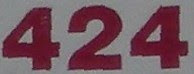On April 10, 1941, USS Niblack (DD 424) used depth charges against a German U-boat while conducing rescue operations of a torpedoed Dutch freighter. This was the first action taken by a U.S. naval vessel against the Axis Powers.

Source: Wikipedia

## Friday, November 19, 2010

### 602

602 = 2 x 7 x 43.

602 is the maximum number of regions into which 25 circles divide the plane.

602 is the smallest number starting a block of five consecutive numbers with the same number of prime divisors (including multiplicity): 602 = 2 x 7 x 43; 603 = 3 x 3 x 67; 604 = 2 x 2 x 51; 605 = 5 x 11 x 11; 606 = 2 x 3 x 101.

602 are the first three digits of 5602.Telephone area code 602 covers most of the city of Phoenix, Arizona.

## Thursday, November 18, 2010

### 901

901 = 17 x 53.

901 has two representations as a sum of two squares: 901 = 12 + 302 = 152 + 262.

901 is the hypotenuse of two primitive Pythagorean triples: 9012 = 602 + 8992 = 4512 + 7802.

901 is the sum of the digits of the first 100 positive integers.901 is a telephone area code in the state of Tennessee.

## Wednesday, November 17, 2010

### 5327

5327 = 7 x 761.

5327 is a multiple of 7 that uses only prime digits (2, 3, 5, and 7).

5327 is a number that cannot be written as a sum of three squares.

5327 is a value of n for which 2n and 7n together use each digit exactly once: 10654 and 37289.Playmobil set #5327 is living room furniture for the Victorian doll house.

## Tuesday, November 16, 2010

### 590

590 = 2 x 5 x 59.

590 plus 590 ones is a prime. This is the only three-digit number with this property.

590 is a number n such that n concatenated with n + 2 gives the product of two numbers that differ by 1: 590592 = 768 x 769.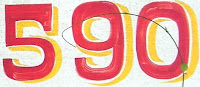590 Tomyris is an asteroid discovered in 1906.

## Monday, November 15, 2010

### 70

70 = 2 x 5 x 7.

The sum of its divisors, including 70 itself, is a square (144): 1 + 2 + 5 + 7 + 10 + 14 + 35 + 70 = 144 = 122.

70 has a unique representation as a sum of three squares: 70 = 32 + 52 + 62.

70 is the 5th pentatope number.

70 is 77 in base 9.Three score years and ten (70) is traditionally considered the natural human life span.

Source: The Penguin Dictionary of Curious and Interesting Numbers, rev. ed., by David Wells.

## Friday, November 12, 2010

### 1271

1271 = 31 x 41.

1271 is a number that cannot be written as a sum of three squares.

1271 has a sixth power whose last few digits are: . . . 21211121.

1271 is the sum of six positive fifth powers: 1271 = 45 + 35 + 15 + 15 + 15 + 15.Marco Polo left Venice in the year 1271 on his travels to Asia.

## Thursday, November 11, 2010

### 168

168 = 23 x 3 x 7.

168 has a unique representation as a sum of three squares: 168 = 22 + 82 + 102.

168 is the number of primes less than 1,000.

144,648 = 861 x 168 = 492 x 294.

168 is the total number of spots on a standard (double-6) set of dominoes.168 is the number of hours in a week.

## Wednesday, November 10, 2010

### 202

202 = 2 x 101.

202 has a representation as a sum of two squares: 202 = 92 + 112.

202 is the sum of four consecutive primes: 202 = 43 + 47 + 53 + 59.

202 is a semiprime palindrome equal to (2 + 3 + 5 + 7)2 - (22 + 32 + 52 + 72).

202293 begins with the digits 293 (a prime). Note that 293202 begins with the digits 202.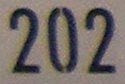202 is the telephone area code for Washington, D.C.

## Tuesday, November 9, 2010

### 580

580 = 22 x 5 x 29.

580 has two representations as a sum of two squares: 580 = 22 + 242 = 162 + 182.

580 is a divisor of 592 - 1.

580 is 2404 in base 6, 404 in base 12, and 202 in base 17.Interstate 580 in northern California connects the San Francisco Bay area with Interstate 5 through California's Central Valley.

## Monday, November 8, 2010

### 812

812 = 22 x 7 x 29. It is the product of a prime and the previous number (two consecutive integers): 812 = 28 x 29.

812 is a pronic number.

812 is the number of triangles of any size contained in a triangle of side 14 on a triangular grid.

The sum of the base 3 digits of 812 equals the sum of the base 9 digits of 812: (1 + 0 + 1 + 0 + 0 + 0 + 2) = (1 + 1 + 0 + 2) = 4.Telephone area code 812 covers the southern part of Indiana.

## Friday, November 5, 2010

### 369

369 = 32 x 41.

369 has a representation as a sum of two squares: 369 = 122 + 152.

369 is the magic constant of an 9-by-9 magic square.

369 is the number of octominoes.369 Aeria is a large asteroid discovered in 1893.

## Thursday, November 4, 2010

### 949

949 = 13 x 73.

949 has two representations as a sum of two squares: 949 = 72 + 302 = 182 + 252.

949 is the hypotenuse of two primitive Pythagorean triples: 9492 = 3012 + 9002 = 4202 + 8512.

949 is the larger number in a Ruth-Aaron pair.NGC 949 is a galaxy in the constellation Triangulum.

## Wednesday, November 3, 2010

### 154

154 = 2 x 7 x 11.

154 is the maximum number of regions into which 17 lines divide the plane.

154 is the smallest number that is palindromic in bases 6 (414), 8 (232), and 9 (181).William Shakespeare wrote 154 sonnets.

## Tuesday, November 2, 2010

### 78

78 = 2 x 3 x 13.

78 has a unique representation as a sum of three squares: 78 = 22 + 52 + 72.

78 is the smallest number with seven representations as a sum of two primes: 78 = 5 + 73 = 7 + 71, 11 + 67 = 17 + 61 = 19 + 59 = 31 + 47 = 37 + 41.

78 is the twelfth triangular number. According to the song "The Twelve Days of Christmas," 78 gifts were sent on the twelfth day.

78 is 66 in base 12.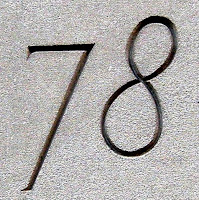A 78 is an old type of gramophone record playing at 78 revolutions per minute.

Source: Phillips, R. 2004. Numbers: Facts, Figures and Fiction. Badsey Publications.

## Monday, November 1, 2010

### 750

750 = 2 x 3 x 53. It is the product of exactly five primes, three of which are distinct (the first three primes).

750 is a number with 16 divisors: 1, 2, 3, 5, 6, 10, 15, 25, 30, 50, 75, 125, 150, 250, 375, 750.The customary size of a wine bottle in the United States is 750 milliliters

## Friday, October 29, 2010

### 262

262 = 2 x 131. It is the product of two distinct Sophie Germain primes.

262 is a meandric number: the number of ways a river can cross a road n times.

The sum of the fourth powers of the prime factors of 262 is a prime: 24 + 1314 = 294499937, a prime.

The concatenation of the proper divisors (excluding 1 and the number itself) of 262 is a prime: 2131 .The Messerschmitt Me 262 was the world's first operational jet-powered fighter aircraft.

Source: Wikipedia

## Thursday, October 28, 2010

### 55

55 = 5 x 11.

55 is the tenth Fibonacci number.

55 is the tenth triangular number.

55 is a pyramidal number, the sum of the first five square numbers: 55 = 12 + 22 + 32 + 42 + 52.

55 is a number that cannot be written as a sum of three squares.An emerald anniversary celebrates 55 years.

Source: Phillips, R. 2004. Numbers: Facts, Figures and Fiction. Badsey Publications.

## Wednesday, October 27, 2010

### 140

140 = 22 x 5 x 7.

140 has a unique representation as a sum of three squares: 140 = 22 + 62 + 102.

140 is a square pyramidal number. It is the sum of the first seven squares: 140 = 12 + 22 + 32 + 42 + 52 + 62 + 72.140 Siwa is a large, dark asteroid discovered in 1874.

## Tuesday, October 26, 2010

### 434

434 = 2 x 7 x 31.

434 is a palindrome that produces a composed prime: 42 + 37 + 431 = 4611686018427390107, a prime.

434 is the smallest composite number that satisfies the equation sigma(n + 2) = sigma(n) + 2, where sigma(n) is the sum of the divisors of n.

434 is 2002 in base 6.434 Hungaria is an asteroid discovered in 1898.

## Monday, October 25, 2010

### 859

859 is a prime number.

857 and 859 form a twin prime pair.

859 is the largest prime that cannot be the central number in a 3-by-3 magic square.

859 is the sum of nine positive fifth powers: 859 = 15 + 15 + 25 + 25 + 25 + 25 + 35 + 35 + 35.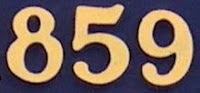859 Bouzar

# é

ah is an asteroid discovered in 1916.

## Friday, October 22, 2010

### 217

217 = 7 x 31.

47 and 89 are primes, as is their concatenation: 4789. Inserting 0 between them transforms the prime into a power: 47089 = 2172.

217 is 1001 in base 6 and 161 in base 12.217 is the telephone area code covering much of central Illinois.

## Thursday, October 21, 2010

### 64

64 = 26.

64 is the first number after 1 to be both a square and a cube: 64 = 82 = 43.

64 is the number of integer partitions of 20 into distinct parts.

64 is 121 in base 7 and 44 in base 15. It is 1000000 in base 2, 1000 in base 4, 100 in base 8, 40 in base 16, and 20 in base 32.The Commodore 64 was an 8-bit home computer introduced in 1982.

Source: Phillips, R. 1994. Number Facts, Figures and Fiction. Cambridge University Press.

## Wednesday, October 20, 2010

### 522

522 = 2 x 32 x 29.

522 has a representation as a sum of two squares: 522 = 92 + 212.

522 is the sum of six consecutive primes: 522 = 73 + 79 + 83 + 898 + 97 + 101.

522 is the sum of seven positive fifth powers: 522 = 15 + 15 + 15 + 15 + 25 + 35 + 35.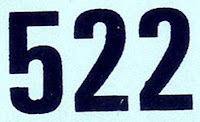The SOKO 522 was a two-seater, Yugoslavian military training and light attack aircraft produced in the 1950s.

Source: Wikipedia

## Tuesday, October 19, 2010

### 703

703 = 19 x 37.

703 is the 37th triangular number. It is also a hexagonal number.

703 is a number that cannot be written as a sum of three squares.

703 is 3131 in base 6.

703 is the smallest number requiring 73 fifth powers for its Waring representation.703 is one of the telephone area codes for northern Virginia.

Source: Wikipedia

## Monday, October 18, 2010

### 422

422 = 2 x 211.

422 is 222 in base 14. It is 646 in base 8.

422 is the maximum number of regions into which 21 circles divide the plane.

422 is the smallest number whose 8th power has 21 digits.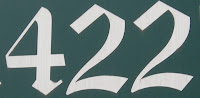422 Berolina is an asteroid discovered in 1896.

## Friday, October 15, 2010

### 100

100 = 22 x 52.

100 has a representation as a sum of two squares: 100 = 62 + 82.

100 is the sum of the first four cubes: 100 = 13 + 23 + 33 + 43. It is the smallest square that is the sum of consecutive cubes.

100 is the sum of the 9th and 10th triangular numbers: 100 = 45 + 55.

100 is the sum of the first 10 odd numbers: 100 = 1 + 3 + 5 + 7 + 9 + 11 + 13 + 15 + 17 + 19.

The first three primes add to 10, and the first 32 primes add to 102 = 100.The Romans wrote 100 as C. This is an abbreviation for the Latin word centum. A cent is a hundredth of a dollar, and a centime was a hundredth of a franc.

## Thursday, October 14, 2010

### 1316

1316 = 22 x 7 x 47.

1316 = 64 + 20.

1316 is 2444 in base 8.

1316 is a number divisible by the sum of the squares of its digits: 12 + 32 + 12 + 62 = 47.

1316 is the Fibonacci number 987 written in base 9.NGC 1316, also known as Fornax A, is a lenticular galaxy about 70 million light-years away in the constellation Fornax.